﻿ 冻融对CACB材料粘弹性力学行为的影响
«上一篇文章快速检索 高级检索

 哈尔滨工程大学学报2019, Vol. 40Issue (8): 1375-1380  DOI: 10.11990/jheu.2018070760

### 引用本文LI Yunliang, SUN Haijiao, LIU Yuze, et al. Effect of freeze-thaw cycles on the viscoelastic mechanical behavior of CACB[J]. Journal of Harbin Engineering University, 2019, 40(8), 1375-1380. DOI: 10.11990/jheu.201807076.### 文章历史

Effect of freeze-thaw cycles on the viscoelastic mechanical behavior of CACB
LI Yunliang, SUN Haijiao , LIU Yuze , TAN Yiqiu , XU Lei , HE Xin
School of Transportation Science and Engineering, Harbin Institute of Technology, Harbin 150090, China
Abstract: The viscoelastic mechanical behavior of cement asphalt composite binder (CACB) under the influence of freeze-thaw cycles was studied through the freeze-thaw and creep tests. A viscoelastic mechanical model of four elements and five parameters was established, and the constitutive equation of CACB was set up on the basis of the fitting analysis of creep test results. The change in constitutive model parameters with freeze-thaw cycles were studied to analyze the laws underlying the influence of freeze-thaw cycles on the viscoelastic mechanical behavior of CACB. This study demonstrated that freeze-thaw cycles weakened the resistance of CACB to instant elastic deformation and shear flow deformation and dramatically influenced the elastic properties of CACB. The change in viscoelastic constitutive model parameters can be used to characterize the general mechanical properties of CACB with freeze-thaw damage. Although freeze-thaw cycles did not change the viscoelasticity of CACB, CACB exhibited viscous-material-like mechanical properties, reduced load resistance, and increased deformability as asphalt content and temperature increased.
Keywords: cement asphalt composite binder    freeze-thaw    creep    viscoelasticity    damage    constitutive equation

1 原材料及试验方案 1.1 CACB原材料及配比表 1 乳化沥青基本性质 Table 1 Fundamental properties of emulsified asphalt表 2 水泥基本性质 Table 2 Fundamental properties of cement表 3 CACB材料配比 Table 3 Mix proportion of CACB
1.2 试验方法

1) 试件制备。

2) 快速冻融试验。

3) 蠕变试验。表 4 蠕变试验方案 Table 4 Scheme of creep test
2 力学模型及本构方程

 $\sigma_{2}=\eta_{2} \varepsilon_{2}^{(r)}$ (1)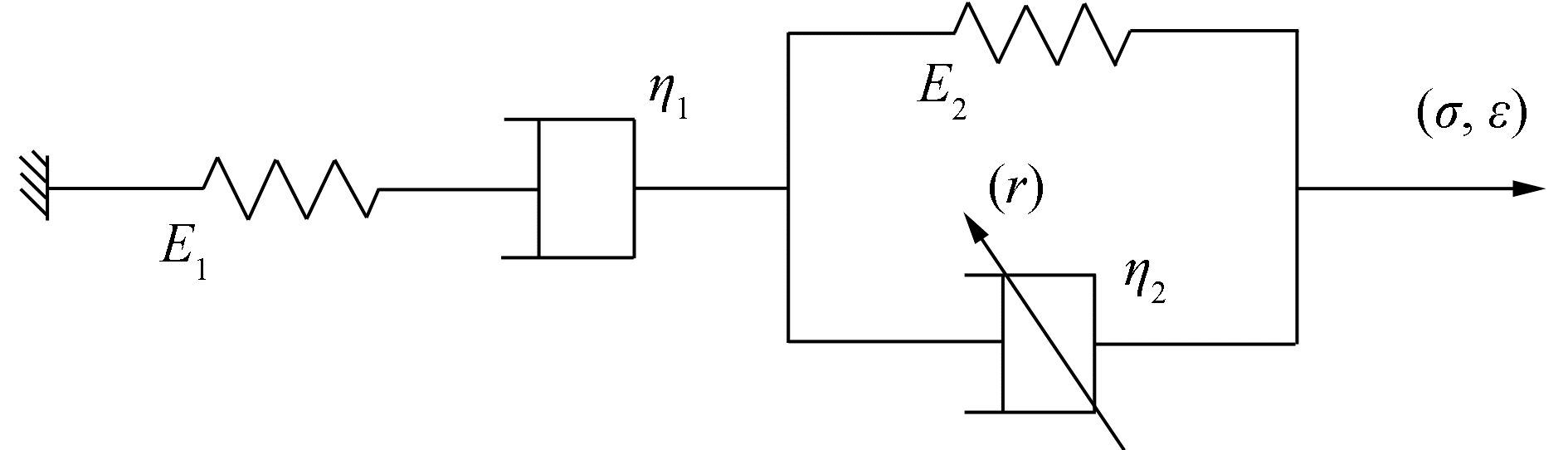Download: 图 1 四元件五参数模型 Fig. 1 Model of four units and five parameters

 $J_{1}(t)=1 / E_{1}$ (2)

 $J_{2}(t)=t / \eta_{1}$ (3)

 $\sigma_{2}=E_{2} \varepsilon_{2}+\eta_{2} \varepsilon_{2}^{(r)}$ (4)

 $\overline{\sigma}_{2}(S)=\left(E_{2}+\eta_{2} S^{r}\right) \overline{\varepsilon}_{2}(S)$ (5)

 $\overline{J}_{3}(S)=1 /\left(E_{2}+\eta_{2} S^{r}\right) S$ (6)

 $J_{3}(t)=\frac{1}{E_{2}} \sum\limits_{1}^{\infty} \frac{(-1)^{n}\left(E_{2} / \eta_{2}\right)^{n+1} t^{(n+1) r}}{\mathit{\Gamma}[(n+1) r+1]}$ (7)

 $J_{3}(t)=\frac{1}{E_{2}} \sum\limits_{1}^{\infty} \frac{\left(-t^{r} E_{2} / \eta_{2}\right)^{n}}{n !}$ (8)

 $\mathrm{e}^{x}=1+x+\frac{x^{2}}{2}+\cdots \frac{x^{n}}{n !}$ (9)

 $x=E_{2} t^{r} / \eta_{2}$ (10)

 $J_{3}(t)=\left(1-\mathrm{e}^{-\frac{R_{2} t^{r}}{\eta_{2}}}\right) / E_{2}$ (11)

 $J(t)=J_{1}(t)+J_{2}(t)+J_{3}(t)$ (12)

 $J(t) = \frac{1}{{{E_1}}} + \frac{1}{{{\eta _1}}} + \frac{1}{{{E_2}}}\left( {1 - {{\rm{e}}^{ - \frac{{{E_2}{t^r}}}{{{\eta _2}}}}}} \right)$ (13)
3 试验结果及参数拟合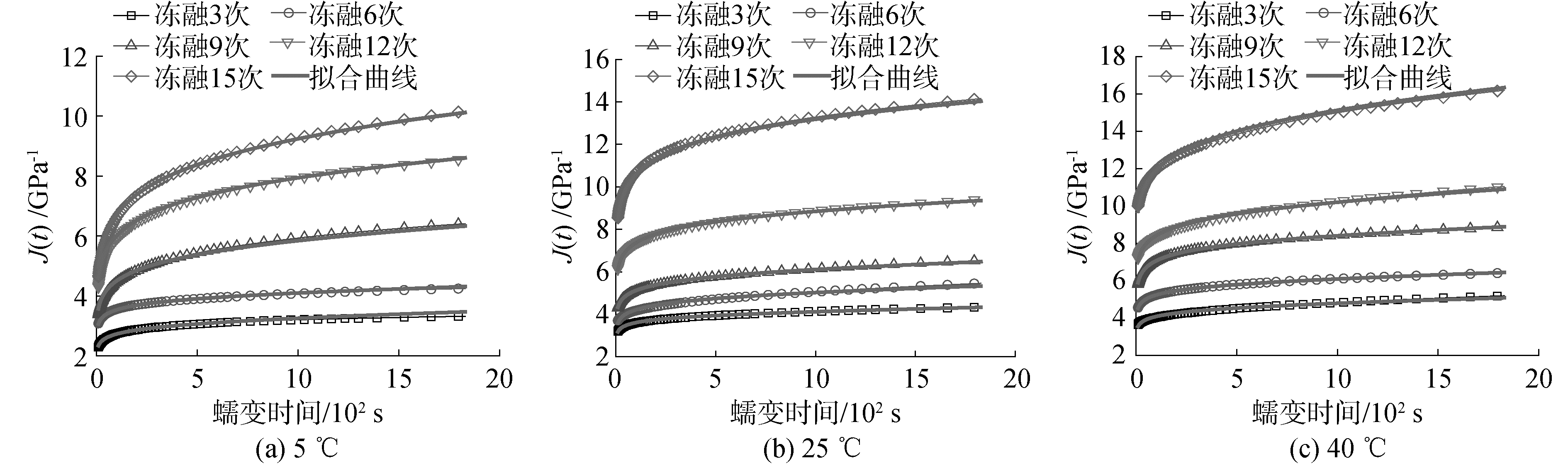Download: 图 2 不同冻融次数下的蠕变柔量曲线(A/C=1.2) Fig. 2 Creep compliance curves at different times of freeze-thaw cycle(A/C=1.2)表 5 蠕变柔量参数拟合结果(A/C=1.0) Table 5 Fitting results of creep compliance parameters (A/C=1.0)表 6 蠕变柔量参数拟合结果(A/C=1.2) Table 6 Fitting result of creep compliance parameters (A/C=1.2)
4 分析与讨论 4.1 冻融对CACB材料粘弹性力学参数的影响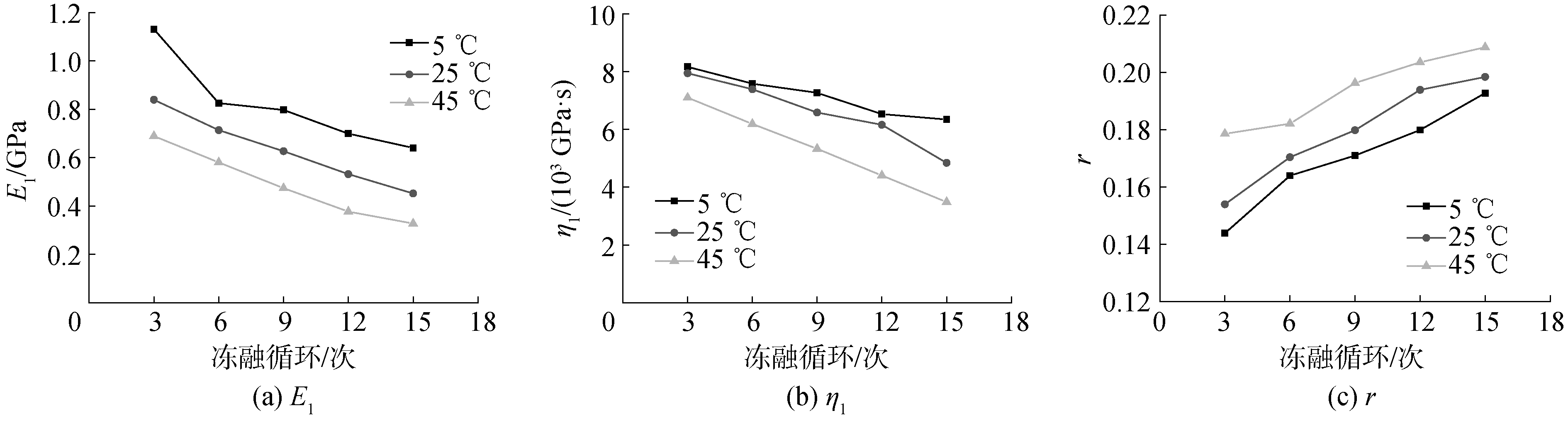Download: 图 3 粘弹性参数随冻融次数的变化(A/C=1.0) Fig. 3 Change of viscoelastic parameters with times of freeze-thaw cycle (A/C=1.0)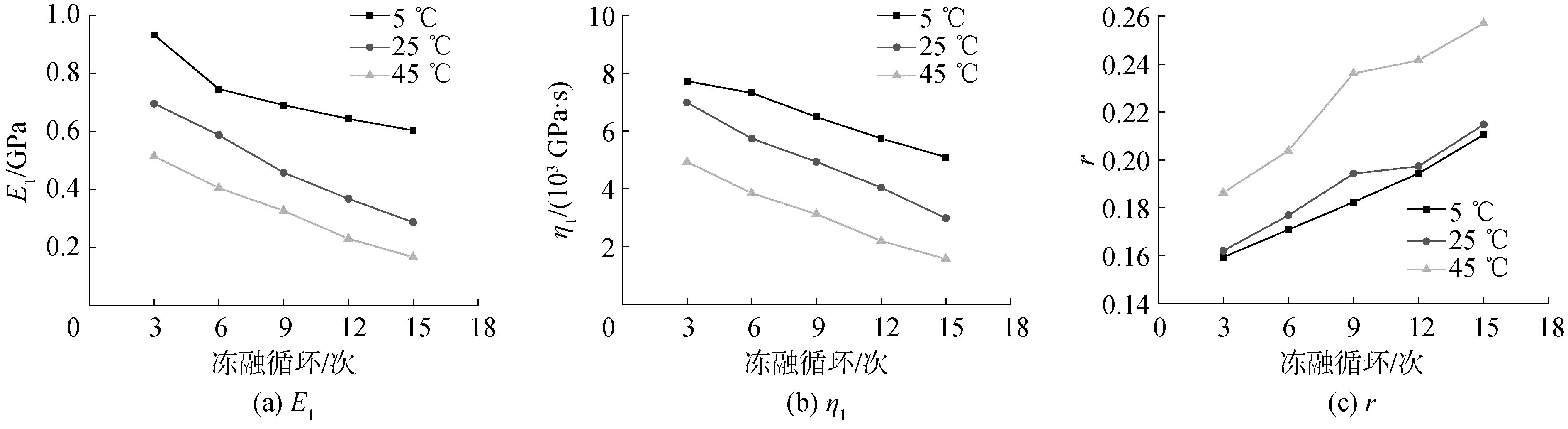Download: 图 4 粘弹性参数随冻融次数的变化(A/C=1.2) Fig. 4 Change of viscoelastic parameters with times of freeze-thaw cycle (A/C=1.2)
4.2 冻融对CACB材料性能损伤的影响

CACB材料的弹性主要由水泥的水化产物所决定，同时沥青的性能及沥青与水泥水化产物的交互作用所形成的微观结构也会影响到CACB材料的弹性性能；而CACB材料的粘性主要由沥青所决定。由于冻融作用会引起CACB材料内部微小的冻融损伤，并且随着冻融循环的持续进行，CACB材料内部的微裂纹会进一步扩展，进而影响到CACB材料的粘弹性性能。冻融作用除了产生冻胀裂纹之外，低温作用也会对沥青的性能产生较大的影响，进而影响到沥青自身的粘弹性性能。由于沥青材料本身具有的粘弹特性，微裂纹对沥青材料性能的影响要小于对水泥材料的影响，因此随着冻胀微裂纹的产生，对CACB材料弹性性能的影响要大于对粘性性能的影响。因此，随着冻融循环的进行，CACB材料的弹性性能及粘性性能均出现了损伤，但弹性性能受冻融的影响更大，相当于冻融作用改变了CACB材料的材料配比，冻融作用导致了CACB材料服役性能的劣化。

4.3 材料配比对CACB材料粘弹性力学参数的影响

CACB材料在材料组成上包括水泥及沥青2种不同力学性质的材料，材料的弹性主要来自水泥的水化产物，粘性主要来自沥青。水泥与沥青的配比不同会影响到材料的粘弹性力学参数。在蠕变试验温度为5 ℃时，不同沥青水泥质量比(A/C)对材料粘弹性力学参数的影响如图 5所示。从图 5可以看出，在不同的冻融循环次数情况下，随着A/C的提高E1η1减小而r增大。可见随着A/C的提高，即沥青用量的增加，胶结料的瞬时弹性模量减少、粘度减小、而粘弹性比例增大，材料更倾向于粘性流体的力学性质。随着沥青用量的增加，材料的强度降低、粘韧性提高，变形能力增强。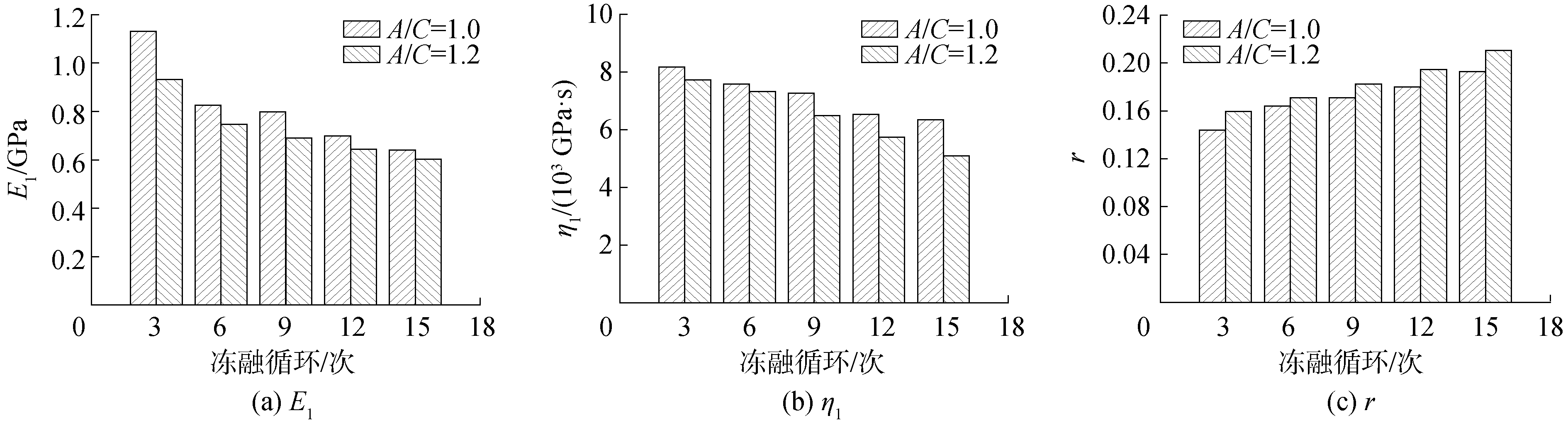Download: 图 5 不同材料配比时的粘弹性参数 Fig. 5 Viscoelastic parameters at different mix proportions
4.4 蠕变试验温度对CACB材料粘弹性力学参数的影响

CACB材料由于沥青的存在，材料呈现较为明显的粘弹特性，温度的变化对材料的粘弹特性的影响较大。图 6为CACB材料粘弹性参数随温度的变化规律。可以看出，在相同的冻融循环次数情况下，E1η1均随温度的升高而减小，r随温度的升高而增大。材料粘弹性力学参数随温度的变化表明，随着温度的升高，材料的瞬时弹性变形、粘性流动变形及延迟弹性变形均呈现增加的趋势。材料粘弹性参数随温度的变化符合典型的粘弹性材料的力学特点。同时通过图 6也可以看出，不同的冻融循环次数并没有改变材料的粘弹性属性，温度对粘弹性参数变化规律的影响基本一致。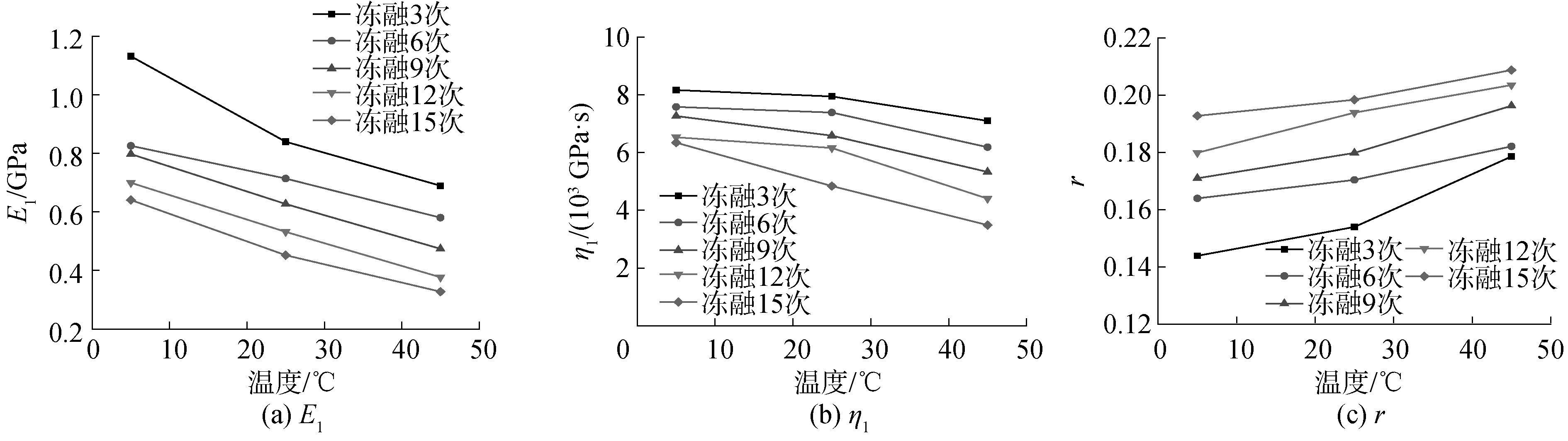Download: 图 6 粘弹性参数随温度的变化(A/C=1.0) Fig. 6 Change of viscoelastic parameters with different temperatures (A/C=1.0)
5 结论

1) 构建了CACB材料的粘弹性本构模型，基于粘弹性力学参数随冻融次数变化的角度，可以较好地分析冻融对CACB材料粘弹性力学性能的影响规律。冻融作用引起CACB材料抵抗瞬时弹性变形及剪切流动变形的能力降低。材料的弹性性能受到冻融的影响更为显著。

2) 随着冻融次数的增加，材料的强度、韧性及减振吸能性能均出现了劣化或改变。本构方程参数随冻融作用的变化规律与材料的宏观损伤特性一致，可以通过E1η1r随冻融次数变化规律的角度分析材料的宏观损伤过程。

3) 冻融循环作用对CACB材料的粘弹性力学行为存在较大的影响，但并不改变CACB材料的粘弹性属性。随着沥青用量的增加或温度的提高，CACB材料抵抗载荷的能力降低、变形能力增强。

  TAN Yiqiu, OUYANG Jian, LYU Jianfu, et al. Effect of emulsifier on cement hydration in cement asphalt mortar[J]. Construction and building materials, 2013, 47: 159-164. DOI:10.1016/j.conbuildmat.2013.04.044 (0)  WANG Yong, YUAN Qiang, DENG Dehua, et al. Measuring the pore structure of cement asphalt mortar by nuclear magnetic resonance[J]. Construction and building materials, 2017, 137: 450-458. DOI:10.1016/j.conbuildmat.2017.01.109 (0)  JIANG Jinyang, SHE Wei, LI Wei, et al. Effect of functional chemical admixtures on the performance of cement asphalt mortar used in ballastless track[J]. Journal of Wuhan University of Technology (materials science edition), 2015, 30(5): 995-1000. DOI:10.1007/s11595-015-1263-x (0)  QIU Kechao, CHEN Huisu, YE Haiping, et al. Thermo-mechanical coupling effect on fatigue behavior of cement asphalt mortar[J]. International journal of fatigue, 2013, 51: 116-120. DOI:10.1016/j.ijfatigue.2013.01.001 (0)  孙东根, 汪波, 朱新实, 等. 水泥乳化沥青碎石基层材料性能与应用[J]. 公路交通科技, 2007, 24(8): 27-31. SUN Donggen, WANG Bo, ZHU Xinshi, et al. Performances and application of cement and emulsified asphalt stabilized macadam[J]. Journal of highway and transportation research and development, 2007, 24(8): 27-31. DOI:10.3969/j.issn.1002-0268.2007.08.007 (0)  耿九光, 陈忠达, 李龙, 等. 水泥-乳化沥青冷再生混合料配合比设计[J]. 长安大学学报(自然科学版), 2009, 29(1): 10-14. GENG Jiuguang, CHEN Zhongda, LI Long, et al. Mixing design of cement-emulsified-asphalt-cold-recycled-mixture (CEACRM)[J]. Journal of Chang'an University (natural science edition), 2009, 29(1): 10-14. DOI:10.3321/j.issn:1671-8879.2009.01.003 (0)  徐媛.乳化沥青改性水泥砂浆在寒冷地区裂缝修补中的应用研究[D].重庆: 重庆交通大学, 2013. XU Yuan. The applied research of emulsified asphalt modified cement mortar on crack repaired in cold areas[D]. Chongqing: Chongqing Jiaotong University, 2013. http://cdmd.cnki.com.cn/article/cdmd-10618-1014377437.htm (0)  李悦, 孙宏友, 曾晓辉, 等. 不同浸水历时及温度对CA砂浆力学性能的影响研究[J]. 铁道标准设计, 2015, 59(2): 40-42. LI Yue, SUN Hongyou, ZENG Xiaohui, et al. Study of the effects of water immersion and temperature on mechanical properties of CA mortar[J]. Railway standard design, 2015, 59(2): 40-42. (0)  王强, 冯竟竟, 阎培渝. 水泥沥青砂浆在室外自然条件下的强度经时损失[J]. 清华大学学报(自然科学版), 2013, 53(1): 1-5, 35. WANG Qiang, FENG Jingjing, YAN Peiyu. Gradual loss of compressive strength of cement asphalt mortar in natural outdoor conditions[J]. Journal of Tsinghua University (science and technology), 2013, 53(1): 1-5, 35. (0)  周锡玲, 谢友均, 郑克仁, 等. 干湿循环作用下水泥乳化沥青砂浆质量变化与体积稳定性[J]. 硅酸盐学报, 2018, 46(7): 895-904. ZHOU Xiling, XIE Youjun, ZHENG Keren, et al. Mass change and volume stability of cement emulsified asphalt mortar in wetting-drying cycles[J]. Journal of the Chinese ceramic society, 2018, 46(7): 895-904. (0)  周锡玲, 郑克仁, 谢友均, 等. 湿含量对水泥乳化沥青砂浆热变形的影响[J]. 建筑材料学报, 2013, 16(5): 817-823, 828. ZHOU Xiling, ZHENG Keren, XIE Youjun, et al. Effect of inner moisture on thermal deformation of cement and emulsified-asphalt mortar[J]. Journal of building materials, 2013, 16(5): 817-823, 828. DOI:10.3969/j.issn.1007-9629.2013.05.014 (0)  田冬梅, 邓德华, 田青, 等. 水对水泥乳化沥青砂浆动态力学性能的影响[J]. 硅酸盐学报, 2013, 41(11): 1507-1513. TIAN Dongmei, DENG Dehua, TIAN Qing, et al. Effect of water on dynamic mechanical properties of cement emulsified asphalt mortar[J]. Journal of the Chinese ceramic society, 2013, 41(11): 1507-1513. (0)  胡曙光, 王涛, 王发洲, 等. CA砂浆抗冻性能的影响因素研究[J]. 武汉理工大学学报, 2008, 30(8): 30-33. HU Shuguang, WANG Tao, WANG Fazhou, et al. Influence factors on freezing and thawing durability of cement asphalt mortar[J]. Journal of Wuhan University of Technology, 2008, 30(8): 30-33. (0)  孔德玉, 毛锦达, 钱晓倩, 等. CRTS Ⅱ型板式无砟轨道CA砂浆抗冻性研究[J]. 建筑材料学报, 2011, 14(6): 859-863. KONG Deyu, MAO Jinda, QIAN Xiaoqian, et al. Frost resistance of CA mortar used in CRTS Ⅱ ballastless slab track[J]. Journal of building materials, 2011, 14(6): 859-863. DOI:10.3969/j.issn.1007-9629.2011.06.027 (0)  毛锦达.板式无碴轨道CA砂浆抗冻与抗疲劳性能研究[D].杭州: 浙江工业大学, 2012. MAO Jinda. Frost and fatigue resistance of CA mortar in ballastless slab track[D]. Hangzhou: Zhejiang University of Technology, 2012. http://cdmd.cnki.com.cn/Article/CDMD-10337-1012514948.htm (0)